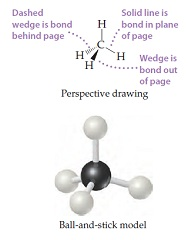# Problem: The methane molecule, CH4, has the geometry shown in following figure. Imagine a hypothetical process in which the methane molecule is "expanded," by simultaneously extending all four C–H bonds to infinity. We then have the process CH4(g) → C(g) + 4 H(g).Suppose that 3.45 g CH4(g) reacts with 1.22 g F2(g), forming CF4(g) and HF(g) as sole products. If the reaction occurs at constant pressure, what amount of heat is evolved?

###### FREE Expert Solution

Balanced reaction: CH4(g) + 4 F2(g) → CF4(g) + 4 HF(g)

ΔH˚rxn = –1952.2 kJ/mol

Find the limiting reactant:

HF from CH4:

molar mass CH4 = 16.042 g/mol

moles HF = 0.860 mol HF

HF from F2:

82% (479 ratings)###### Problem Details
The methane molecule, CH4, has the geometry shown in following figure. Imagine a hypothetical process in which the methane molecule is "expanded," by simultaneously extending all four C–H bonds to infinity. We then have the process CH4(g) → C(g) + 4 H(g).Suppose that 3.45 g CH4(g) reacts with 1.22 g F2(g), forming CF4(g) and HF(g) as sole products. If the reaction occurs at constant pressure, what amount of heat is evolved?

Frequently Asked Questions

What scientific concept do you need to know in order to solve this problem?

Our tutors have indicated that to solve this problem you will need to apply the Enthalpy of Formation concept. You can view video lessons to learn Enthalpy of Formation. Or if you need more Enthalpy of Formation practice, you can also practice Enthalpy of Formation practice problems.